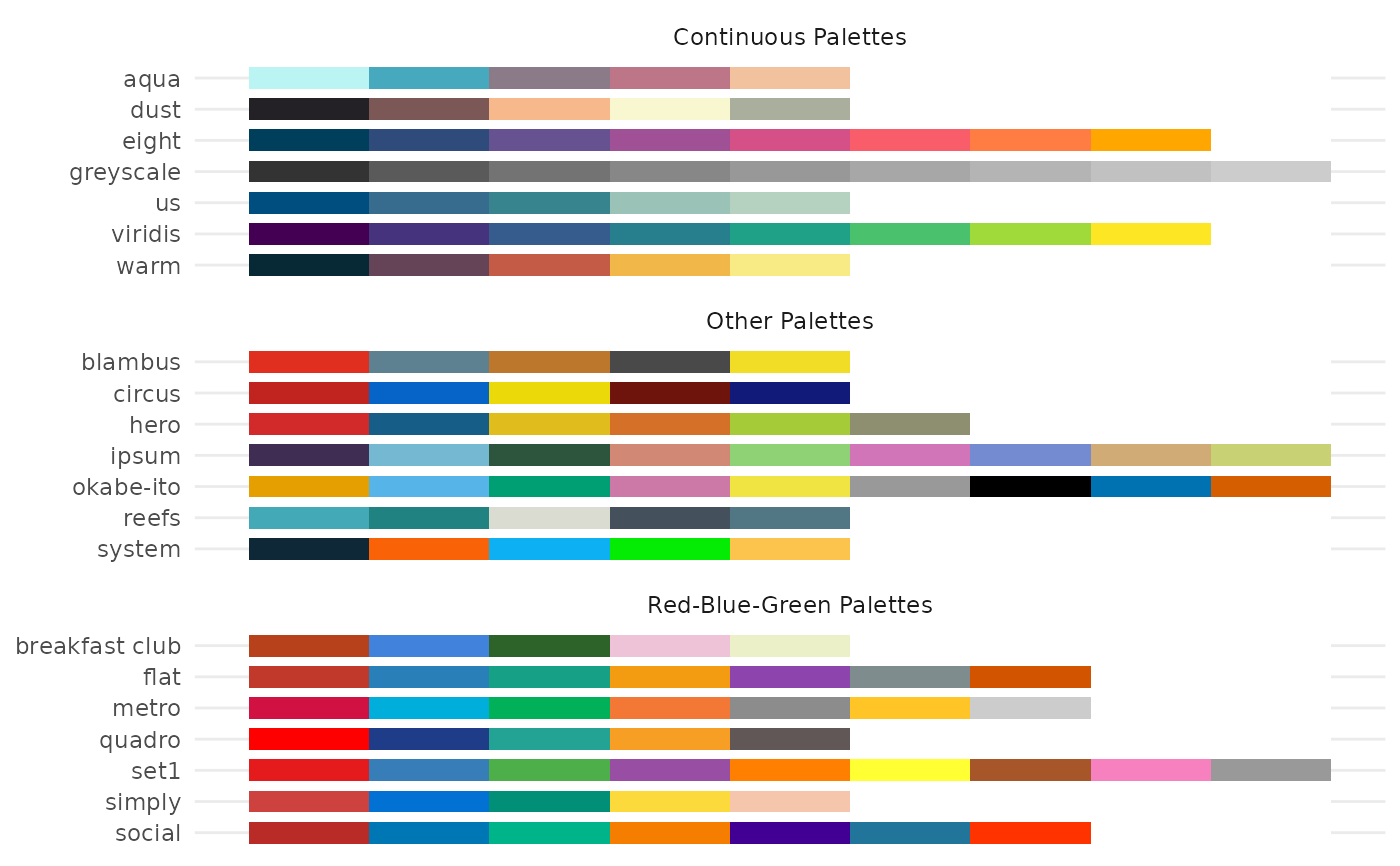A generic plot-method for ggeffects-objects.

## Usage

# S3 method for ggeffects
plot(
x,
show_ci = TRUE,
ci_style = c("ribbon", "errorbar", "dash", "dot"),
show_data = FALSE,
show_residuals = FALSE,
show_residuals_line = FALSE,
data_labels = FALSE,
limit_range = FALSE,
collapse_group = FALSE,
show_legend = TRUE,
show_title = TRUE,
show_x_title = TRUE,
show_y_title = TRUE,
case = NULL,
colors = NULL,
alpha = 0.15,
dot_alpha = 0.35,
jitter = NULL,
dodge = 0.25,
dot_size = NULL,
line_size = NULL,
use_theme = TRUE,
log_y = FALSE,
connect_lines = FALSE,
facets,
grid,
one_plot = TRUE,
verbose = TRUE,
ci = show_ci,
ci.style = ci_style,
rawdata = show_data,
residuals = show_residuals,
residuals.line = show_residuals_line,
label.data = data_labels,
limit.range = limit_range,
collapse.group = collapse_group,
dot.alpha = dot_alpha,
dot.size = dot_size,
line.size = line_size,
connect.lines = connect_lines,
show.title = show_title,
show.x.title = show_x_title,
show.y.title = show_y_title,
use.theme = use_theme,
show.legend = show_legend,
one.plot = one_plot,
log.y = log_y,
...
)

theme_ggeffects(base_size = 11, base_family = "")

show_pals()

## Arguments

x

An object of class ggeffects, as returned by the functions from this package.

show_ci

Logical, if TRUE, confidence bands (for continuous variables at x-axis) resp. error bars (for factors at x-axis) are plotted.

ci_style

Character vector, indicating the style of the confidence bands. May be either "ribbon", "errorbar", "dash" or "dot", to plot a ribbon, error bars, or dashed or dotted lines as confidence bands.

show_data

Logical, if TRUE, a layer with raw data from response by predictor on the x-axis, plotted as point-geoms, is added to the plot.

show_residuals

Logical, if TRUE, a layer with partial residuals is added to the plot. See vignette Effect Displays with Partial Residuals. from effects for more details on partial residual plots.

show_residuals_line

Logical, if TRUE, a loess-fit line is added to the partial residuals plot. Only applies if residuals is TRUE.

data_labels

Logical, if TRUE and row names in data are available, data points will be labelled by their related row name.

limit_range

Logical, if TRUE, limits the range of the prediction bands to the range of the data.

collapse_group

For mixed effects models, name of the grouping variable of random effects. If collapse_group = TRUE, data points "collapsed" by the first random effect groups are added to the plot. Else, if collapse_group is a name of a group factor, data is collapsed by that specific random effect. See collapse_by_group() for further details.

show_legend

Logical, shows or hides the plot legend.

show_title

Logical, shows or hides the plot title-

show_x_title

Logical, shows or hides the plot title for the x-axis.

show_y_title

Logical, shows or hides the plot title for the y-axis.

case

Desired target case. Labels will automatically converted into the specified character case. See ?sjlabelled::convert_case for more details on this argument.

colors

Character vector with color values in hex-format, valid color value names (see demo("colors")) or a name of a ggeffects-color-palette.

Following options are valid for colors:

• If not specified, the color brewer palette "Set1" will be used.

• If "gs", a greyscale will be used.

• If "bw", the plot is black/white and uses different line types to distinguish groups.

• There are some pre-defined color-palettes in this package that can be used, e.g. colors = "metro". See show_pals() to show all available palettes.

• Else specify own color values or names as vector (e.g. colors = c("#f00000", "#00ff00")).

alpha

Alpha value for the confidence bands.

dot_alpha

Alpha value for data points, when show_data = TRUE.

jitter

Numeric, between 0 and 1. If not NULL and show_data = TRUE, adds a small amount of random variation to the location of data points dots, to avoid overplotting. Hence the points don't reflect exact values in the data. May also be a numeric vector of length two, to add different horizontal and vertical jittering. For binary outcomes, raw data is not jittered by default to avoid that data points exceed the axis limits.

dodge

Value for offsetting or shifting error bars, to avoid overlapping. Only applies, if a factor is plotted at the x-axis (in such cases, the confidence bands are replaced by error bars automatically), or if ci_style = "errorbars".

dot_size

Numeric, size of the point geoms.

line_size

Numeric, size of the line geoms.

use_theme

Logical, if TRUE, a slightly tweaked version of ggplot's minimal-theme, theme_ggeffects(), is applied to the plot. If FALSE, no theme-modifications are applied.

log_y

Logical, if TRUE, the y-axis scale is log-transformed. This might be useful for binomial models with predicted probabilities on the y-axis.

connect_lines

Logical, if TRUE and plot has point-geoms with error bars (this is usually the case when the x-axis is discrete), points of same groups will be connected with a line.

facets, grid

Logical, defaults to TRUE if x has a column named facet, and defaults to FALSE if x has no such column. Set facets = TRUE to wrap the plot into facets even for grouping variables (see 'Examples'). grid is an alias for facets.

one_plot

Logical, if TRUE and x has a panel column (i.e. when four terms were used), a single, integrated plot is produced.

verbose

Logical, toggle warnings and messages.

ci, add.data, rawdata, residuals, residuals.line, label.data, limit.range, collapse.group, dot.alpha, dot.size, line.size, connect.lines, show.title, show.x.title, show.y.title, use.theme, one.plot, ci.style, show.legend, log.y

Deprecated arguments. Use show_ci, show_data, show_residuals, show_residuals_line, data_labels, limit_range, collapse_group, dot_alpha, dot_size, line_size, connect_lines, show_title, show_x_title, show_y_title, use_theme, ci_style, show_legend, log_y and one_plot instead.

...

Further arguments passed down to ggplot::scale_y*(), to control the appearance of the y-axis.

base_size

Base font size.

base_family

Base font family.

## Value

A ggplot2-object.

## Details

For proportional odds logistic regression (see ?MASS::polr) or cumulative link models in general, plots are automatically facetted by response.level, which indicates the grouping of predictions based on the level of the model's response.

## Note

Load library(ggplot2) and use theme_set(theme_ggeffects()) to set the ggeffects-theme as default plotting theme. You can then use further plot-modifiers, e.g. from sjPlot, like legend_style() or font_size() without losing the theme-modifications.

There are pre-defined colour palettes in this package. Use show_pals() to show all available colour palettes.

## Partial Residuals

For generalized linear models (glms), residualized scores are computed as inv.link(link(Y) + r) where Y are the predicted values on the response scale, and r are the working residuals.

For (generalized) linear mixed models, the random effect are also partialled out.

## Examples

library(sjlabelled)
data(efc)
efc$c172code <- as_label(efc$c172code)
fit <- lm(barthtot ~ c12hour + neg_c_7 + c161sex + c172code, data = efc)

dat <- ggpredict(fit, terms = "c12hour")
plot(dat)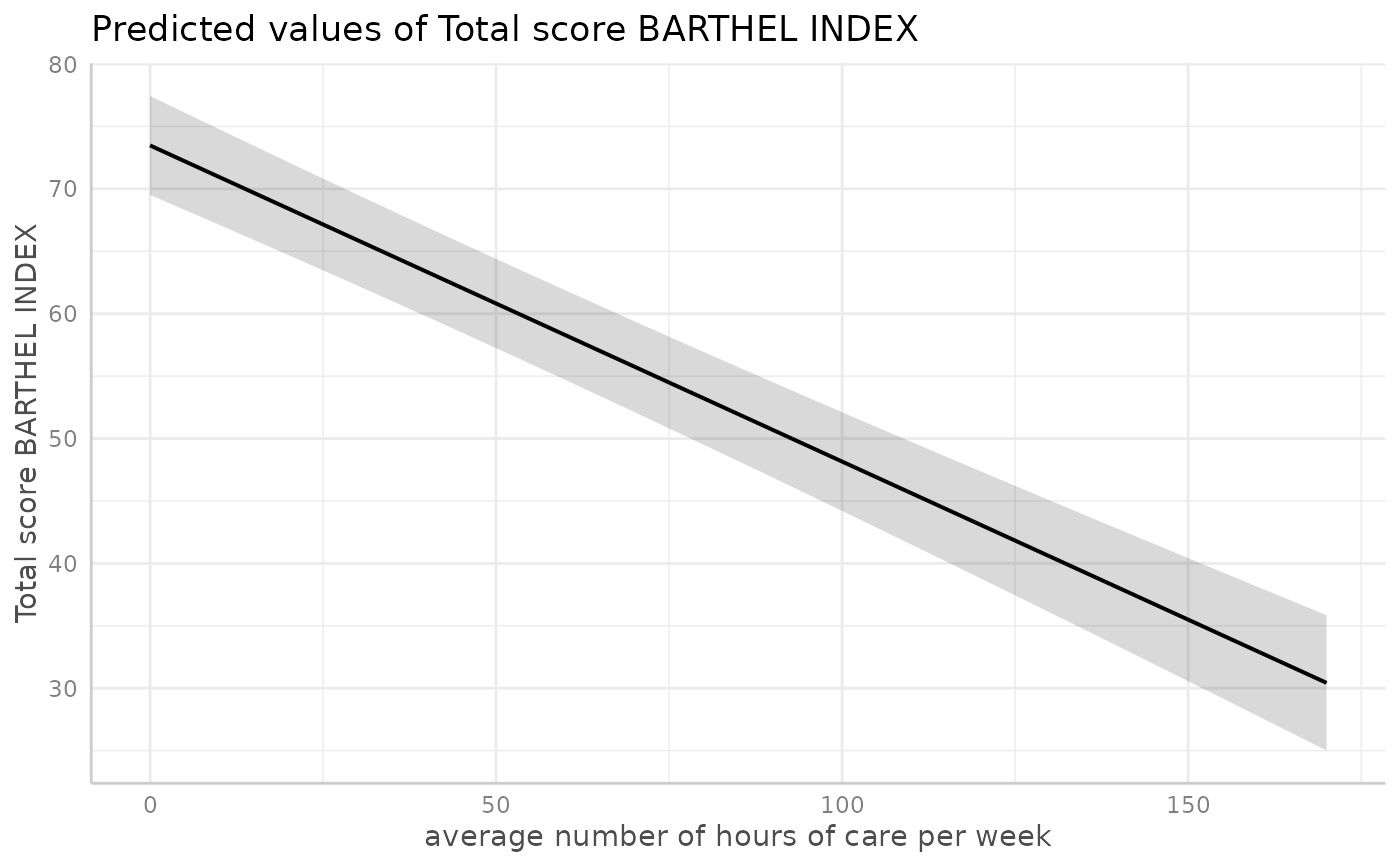# \donttest{
# facet by group, use pre-defined color palette
dat <- ggpredict(fit, terms = c("c12hour", "c172code"))
plot(dat, facet = TRUE, colors = "hero")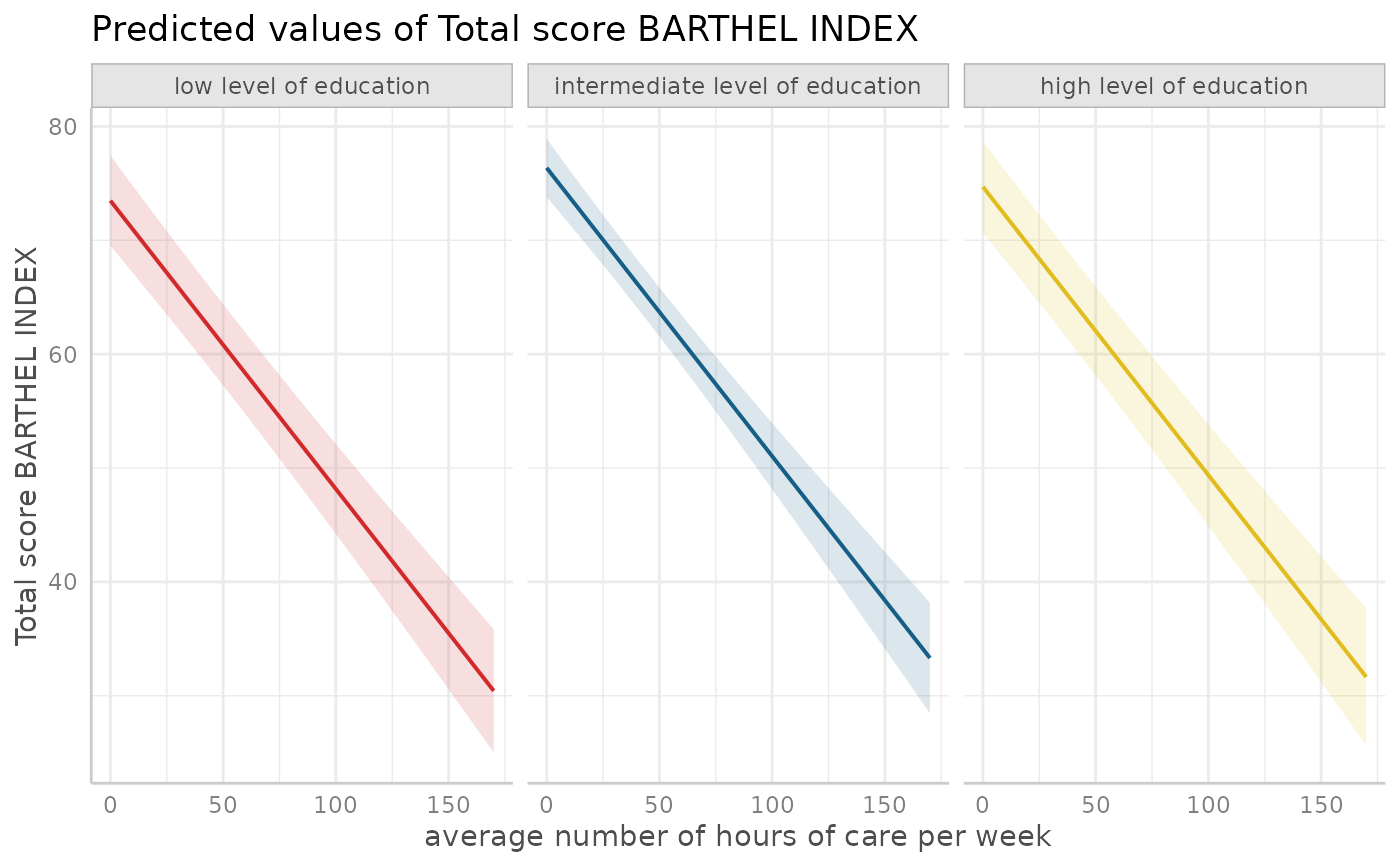# don't use facets, b/w figure, w/o confidence bands
dat <- ggpredict(fit, terms = c("c12hour", "c172code"))
plot(dat, colors = "bw", show_ci = FALSE)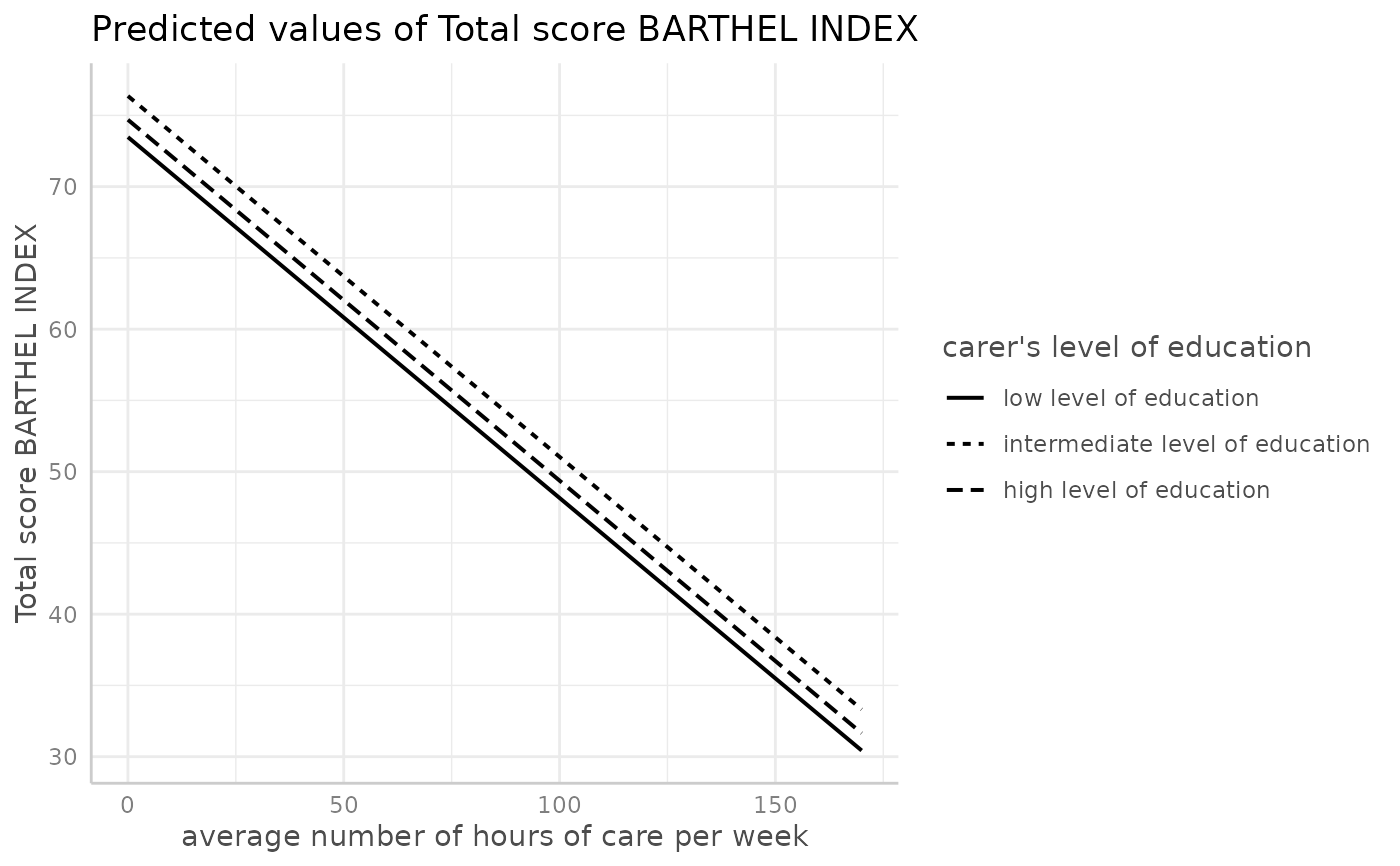# factor at x axis, plot exact data points and error bars
dat <- ggpredict(fit, terms = c("c172code", "c161sex"))
plot(dat)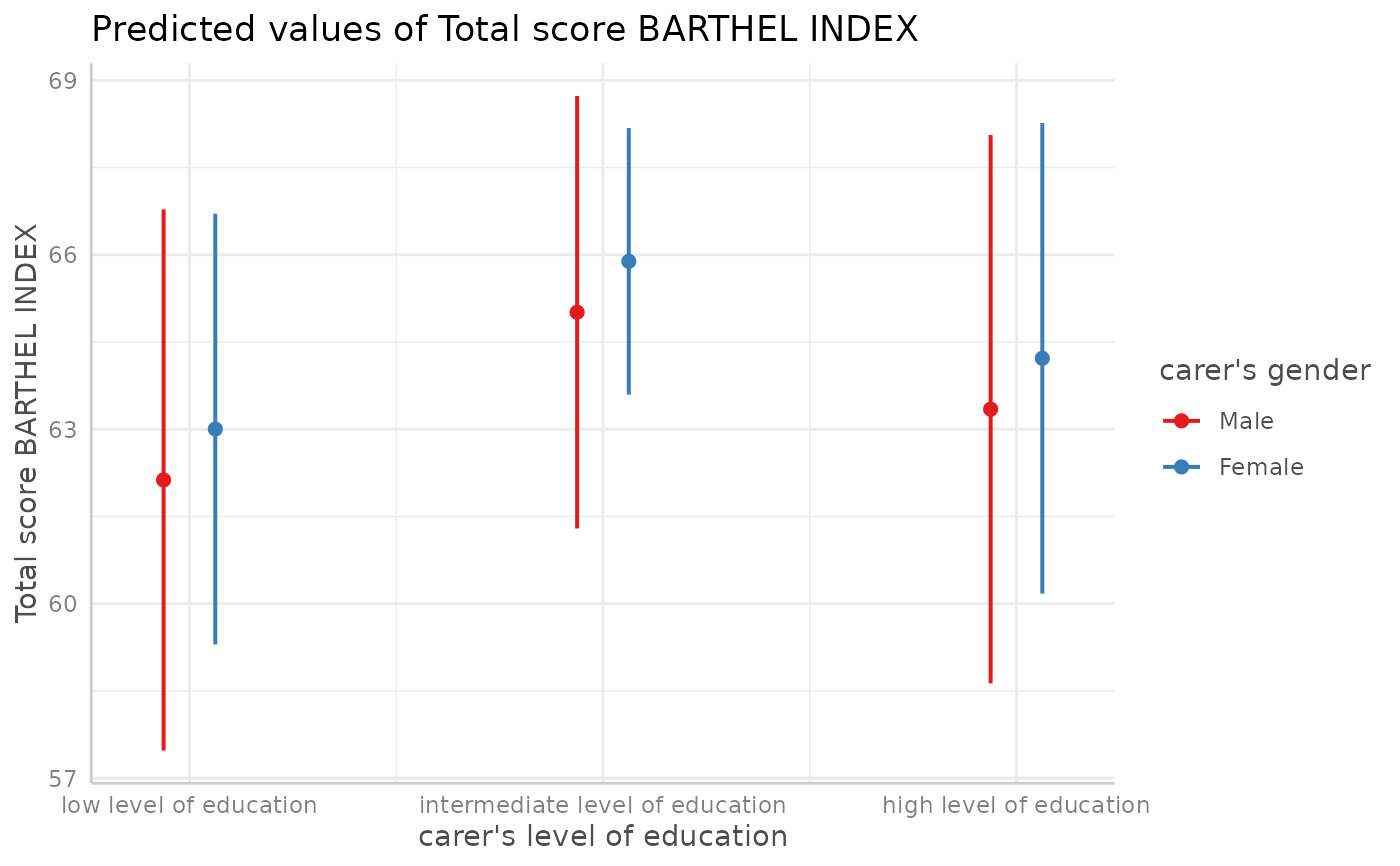# for three variables, automatic facetting
dat <- ggpredict(fit, terms = c("c12hour", "c172code", "c161sex"))
plot(dat)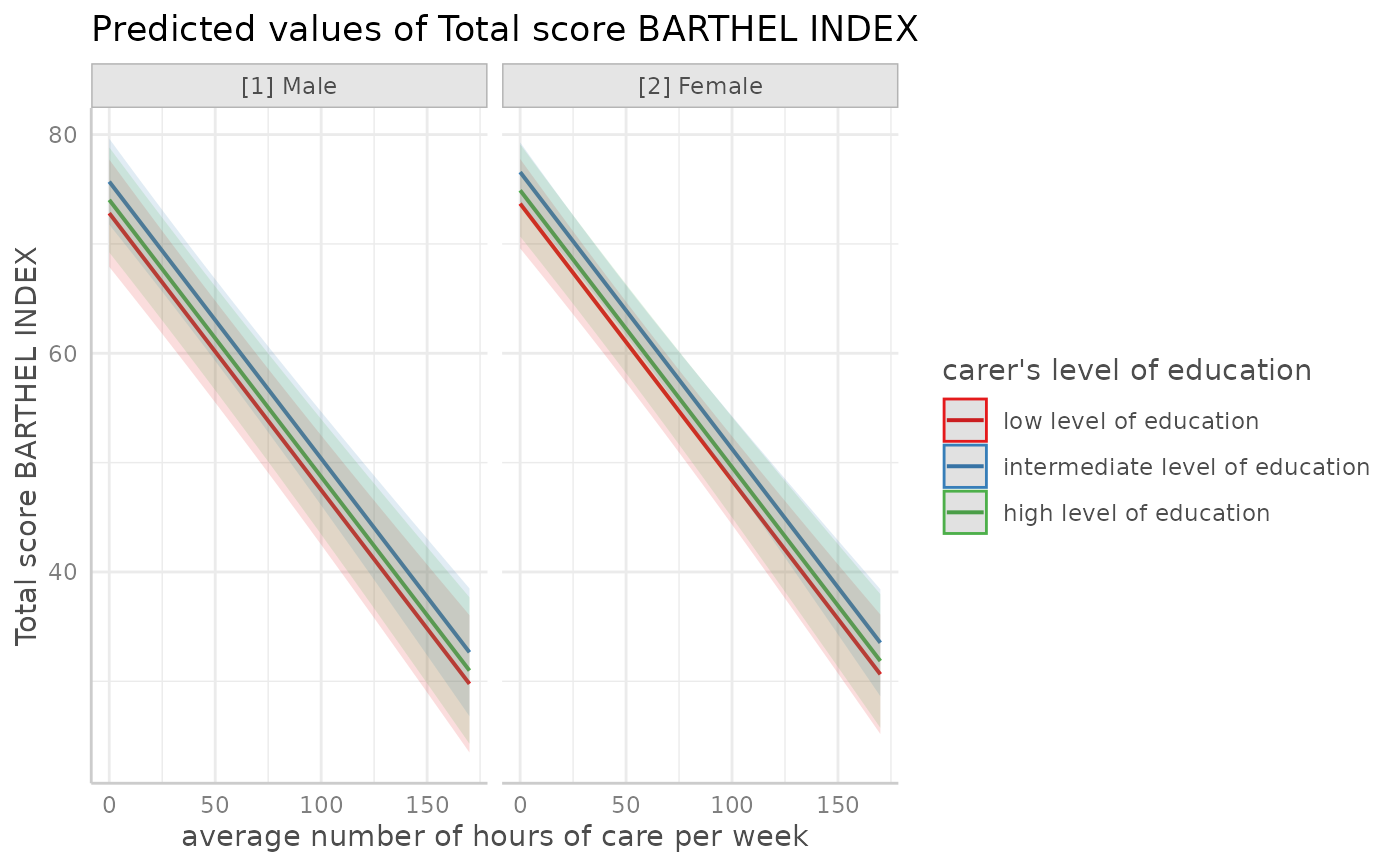# }

# show all color palettes
show_pals()